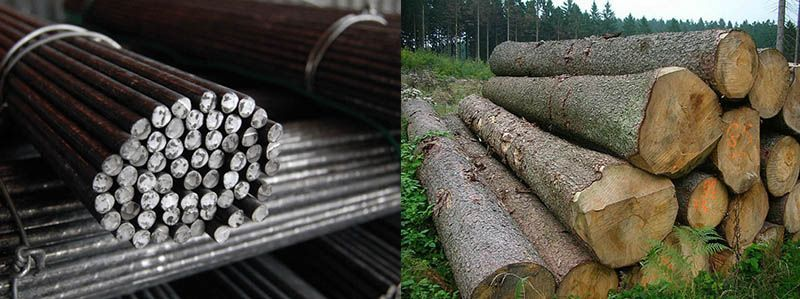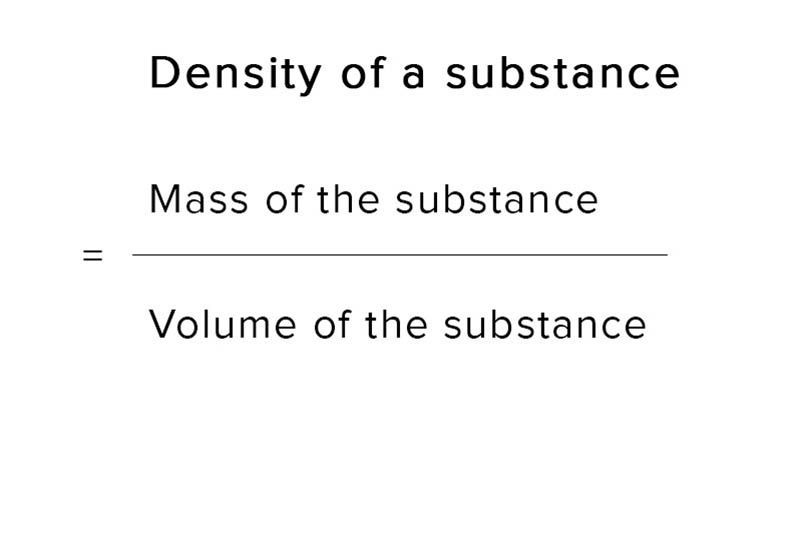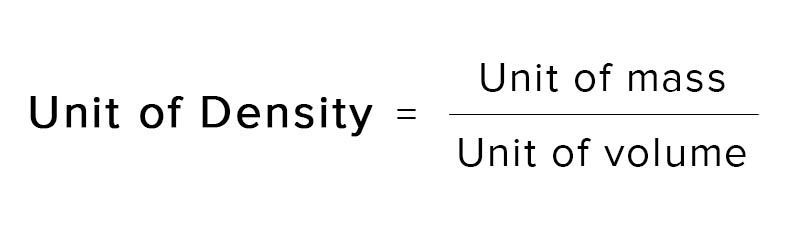# Density

Each body has a certain mass and a volume also. The volume occupied by increases, if its mass is increased. it is found that for:Equal masses of different substances have different volumes. For example, the volume of cotton is much larger than the volume of an equal mass of lead. This is because the particles of lead are closely packed while those of the cotton are very loosely packed. In other words, lead is denser than the cotton.Equal volumes of different substances have different masses. For example, the mass of iron is much more than the mass of an equal volume of wood. This is because the particles of iron are more closely packed than those of the wood. to other words, iron is denser than wood.

# Formula

Thus to explain that equal volumes of (different substances have different masses or equal masses of different substances have different volumes, we use a term called density. It is defined as followsThe density of a substance is its represented by the symbol d.

If mass of a substance is M and its volume is V, its density will be d = M/V.

# Unit of DensityS.I. System

In the S.I. system, unit of mass is kg and unit of volume is m3, so S.I. unit of density is kg m-3.C.G.S. System

In C.G.S. system unit of mass is g and unit of volume is cm3, so CGS unit of density is g cm-3 (gram per cubic centimetre).

# Access All Content

## More from Grade 8 Physics# Heating Effect of Electric Current

Understand the concept of heating effect of electric current. See real world examples where this effect is observed.# Magnetic Effect of Electric Current

Understand the concept of magnetic effect of electric current. Demystify this seemingly magical effect. See it's application in the real world.# Electric Circuit

Understand the basic components of a electrical circuit using an exciting video of RC car.